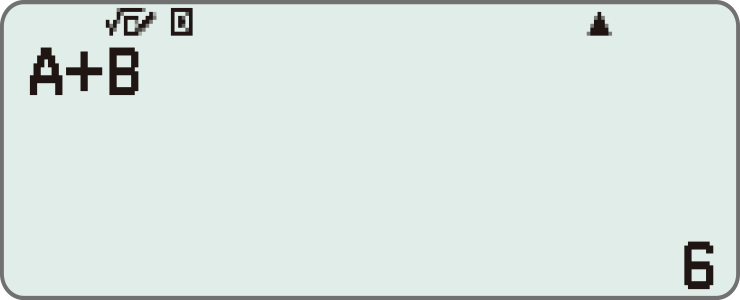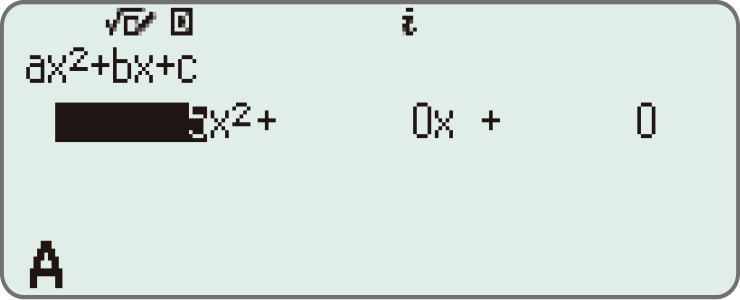# fx-991CW | CASIO

Search

ClassWiz
Standard Scientific Calculators

# fx-991CW

NEW
My GShock

ClassWiz CW Series is designed teaching and studying for today. Its brand-new approachable UI and various features encourage students’ math learning.

## Intuitive, approachable new design

With the 4-gradation display, the current input location is　displayed in a darker color.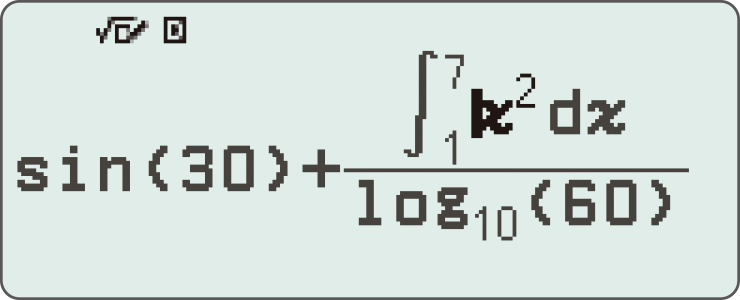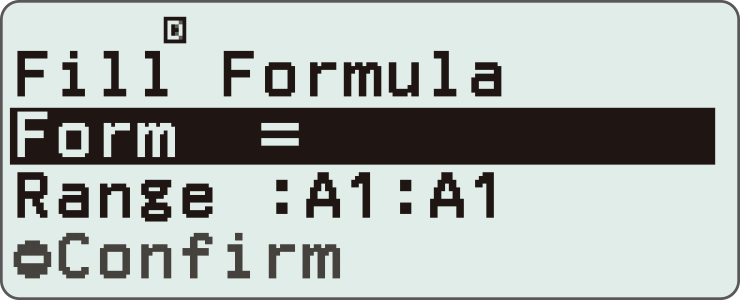Intuitive cursor operation

Simple cursor-based operations and a key layout that follows the natural flow of use.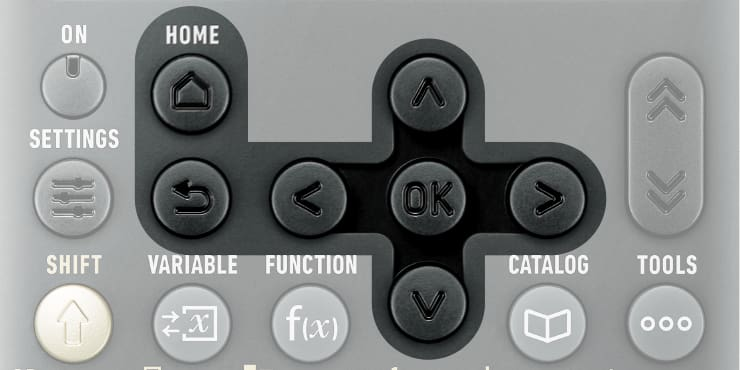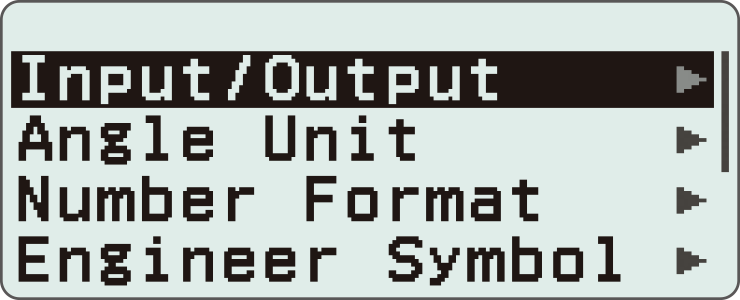Natural Textbook Display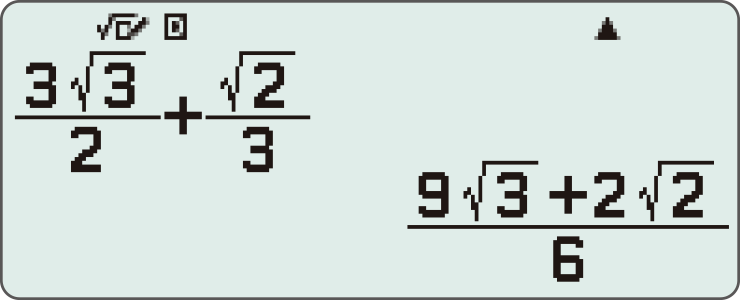Intuitive, easy-to-learn icon display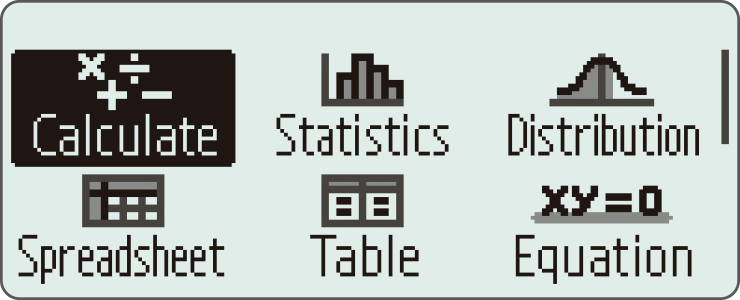List display function for thorough, speedy learning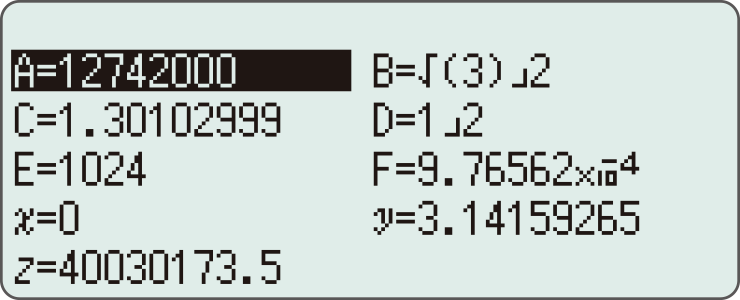High-speed calculation

Fast calculation for smooth performance in class or during examinations.

## Calculator Apps

Easily select a calculator app from the Home screen that is suitable for the type of calculation that you want to perform.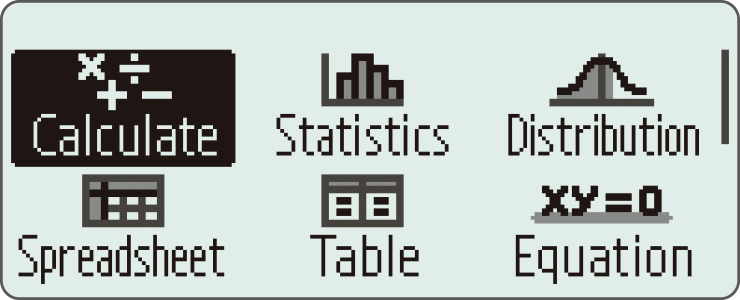##### *The image is the display of fx-991CW.

Useful for learning statistics, recursive formulas, and more.
Handles up to 5 columns x 45 rows (maximum of 170 data items).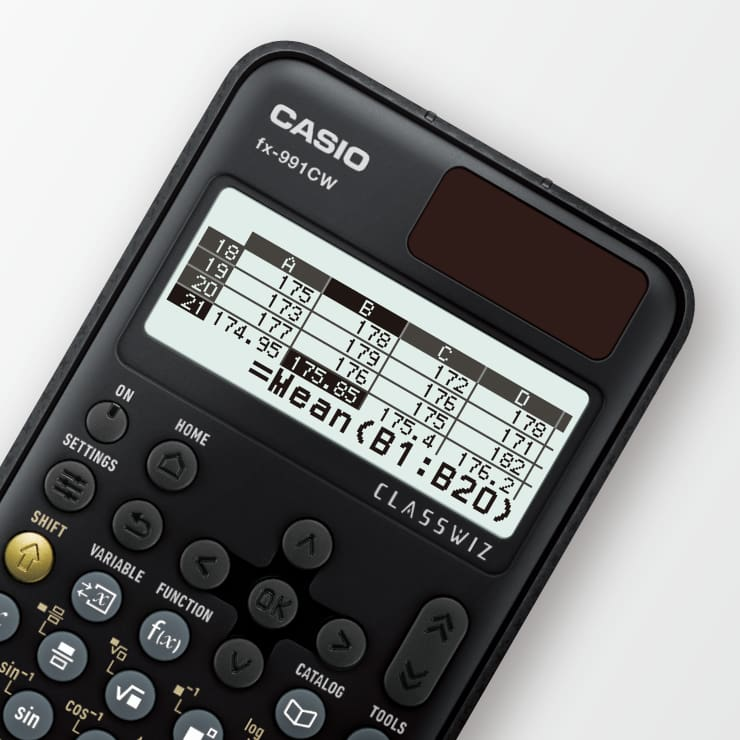Math Box

The following functions to support math learning: Dice Roll and Coin Toss for probability simulations.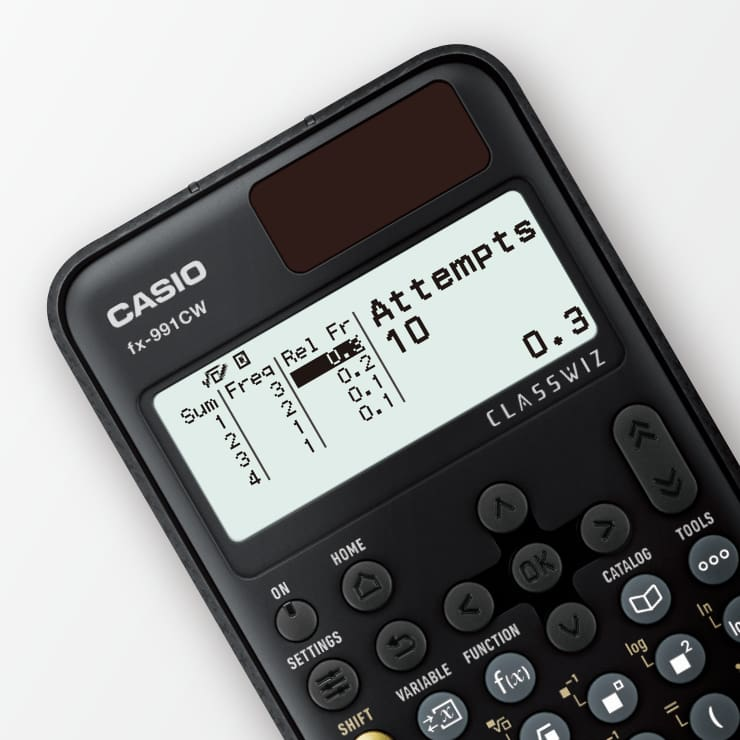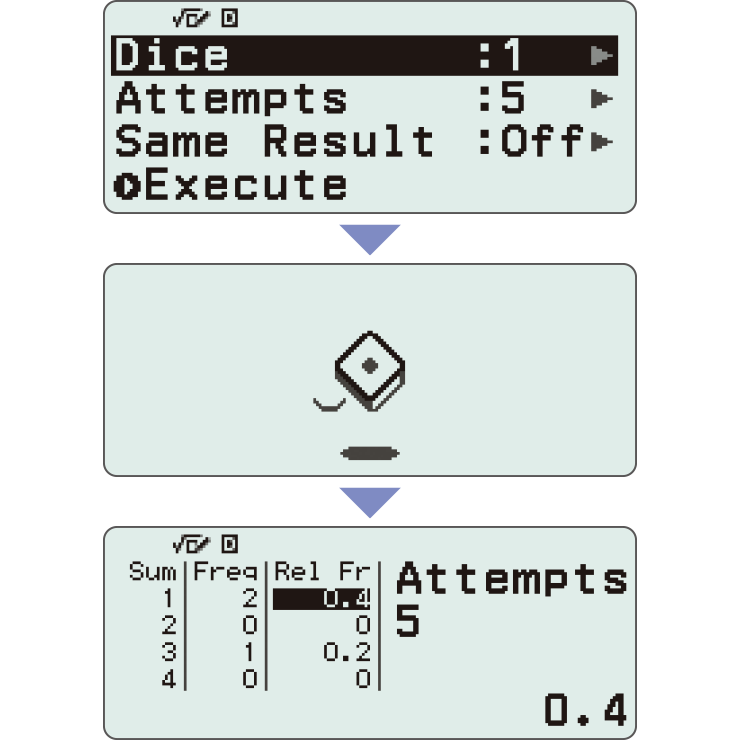##### *Screen images might differ from the final product.

Calculate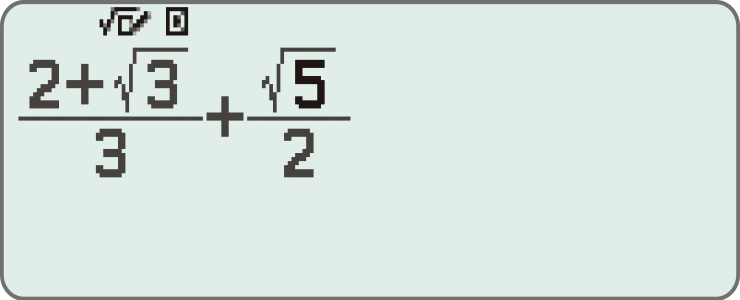General calculations

Statistics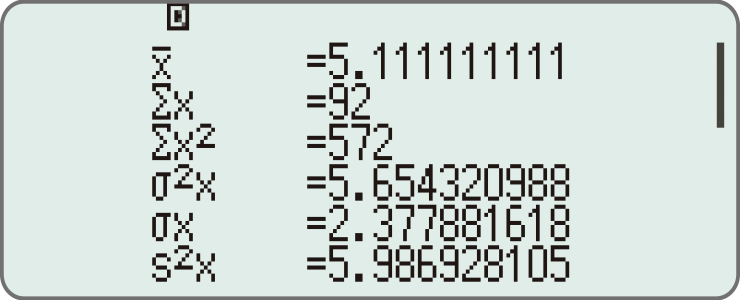Statistical and regression calculations

Table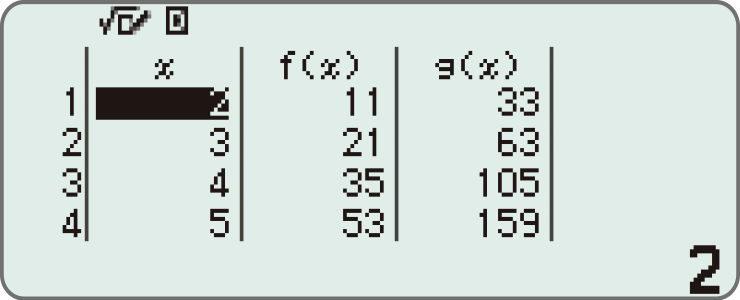Generates a number table based on one or two functions

Equation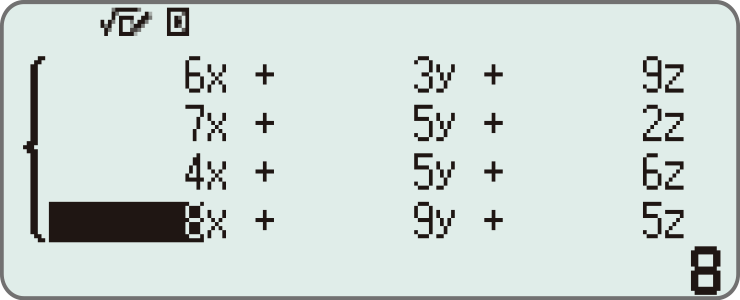Simultaneous equations, higher-order equations, and Solver (function to find the value of any variable contained in an input equation)

## Online visualization service using QR codes

Easily generate QR codes of equations input into the calculator. Graphs and other graphics can be displayed on smartphones or tablets.

1. Input data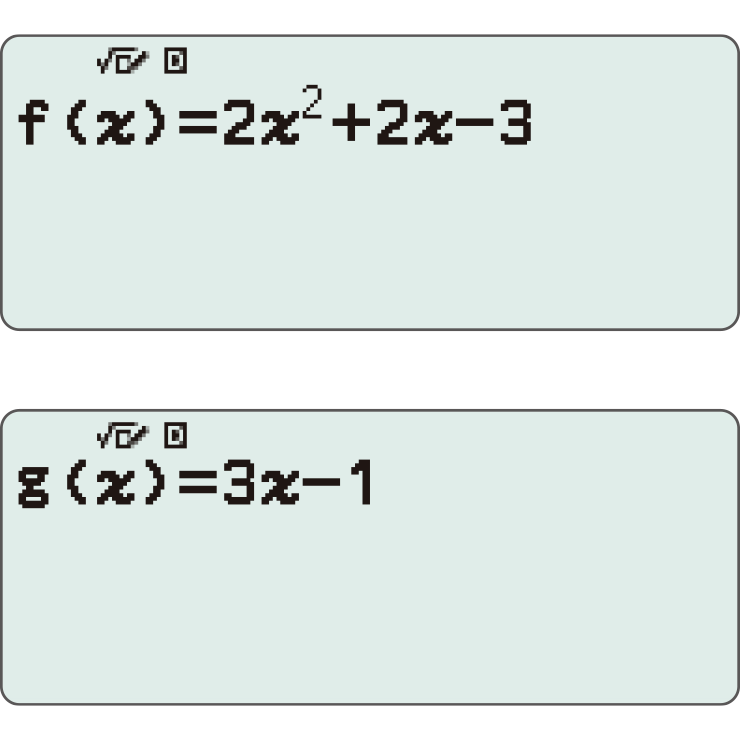2. Generate & scan QR code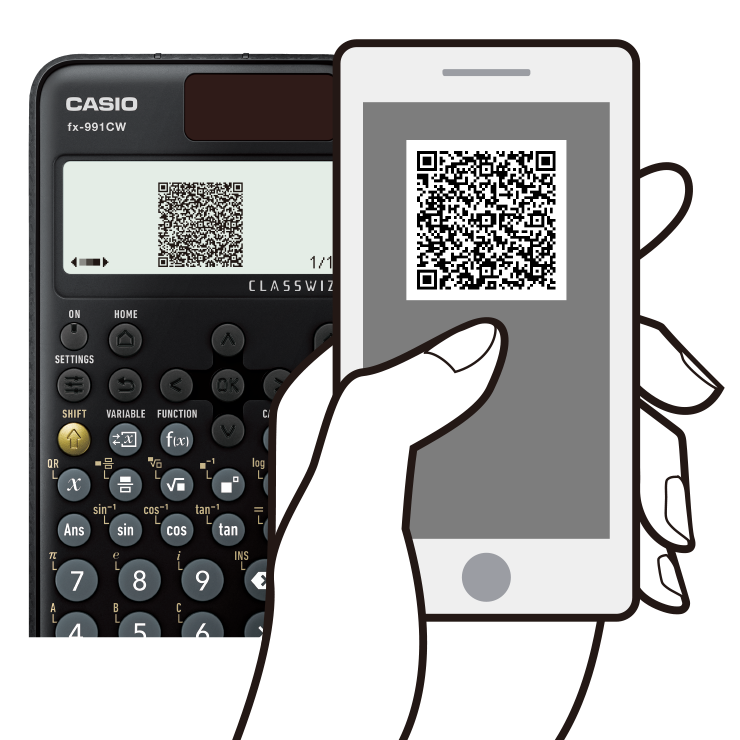3. Graph on smart device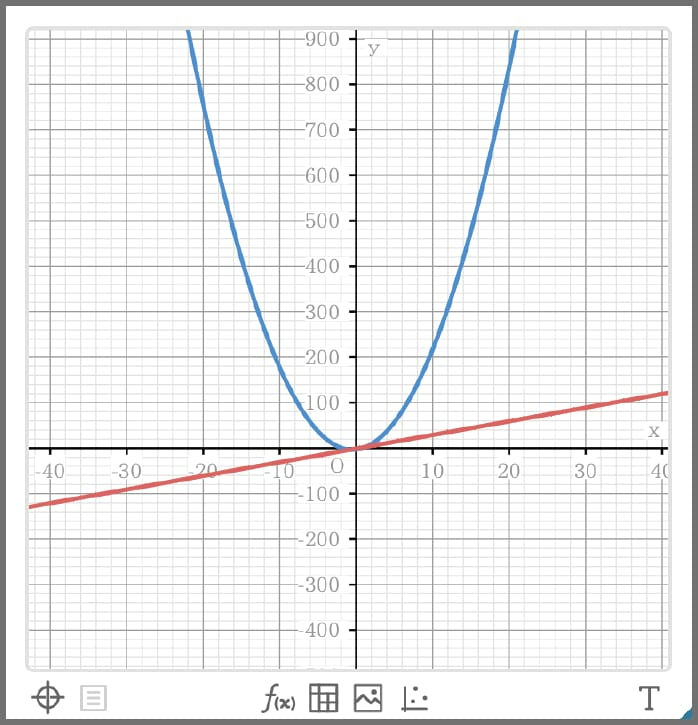4. Analyze graph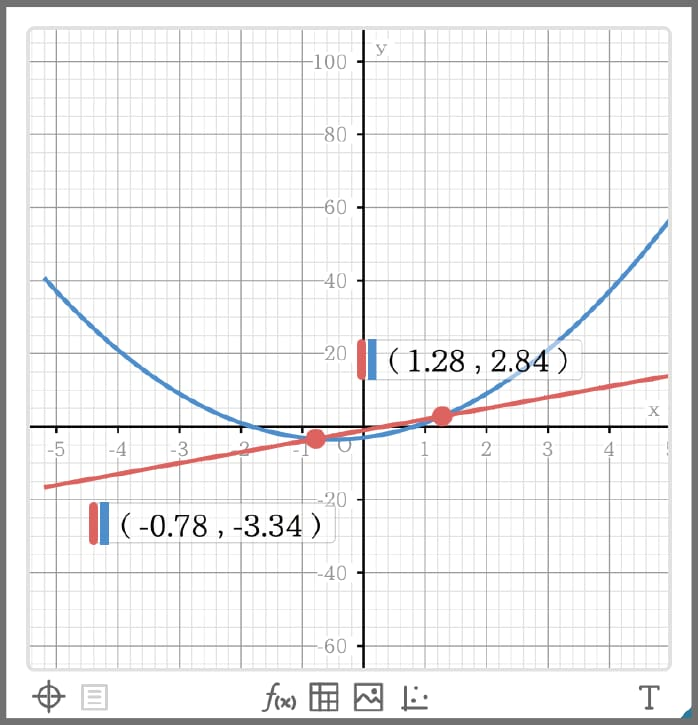## Variety of functions that are available in calculator apps to support students’ learning.

#### CATALOG#### The CATALOG menu shows Store values to variables categories of commands, functions, and symbols according to the calculator app that you are currently using and the current status of the app (the displayed screen or current settings).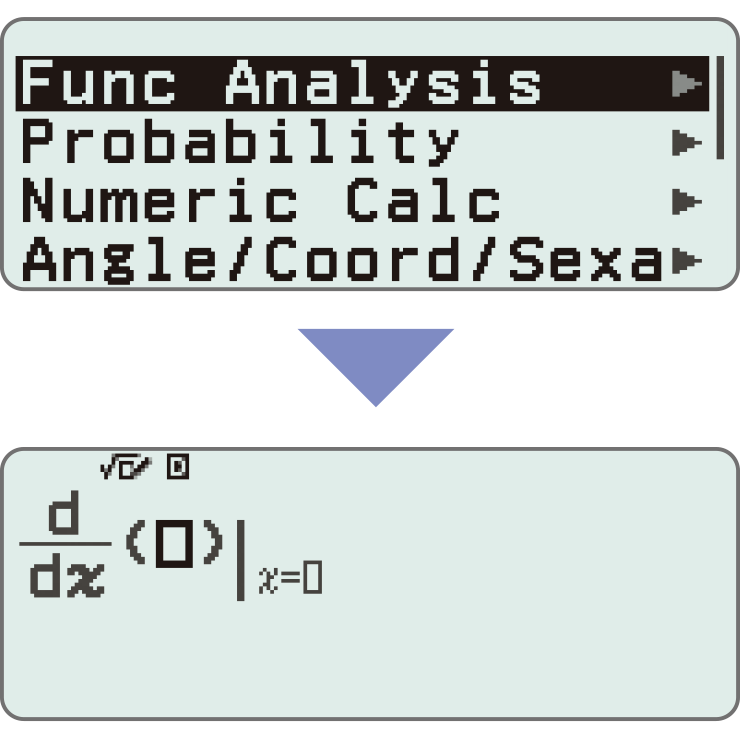#### FUNCTION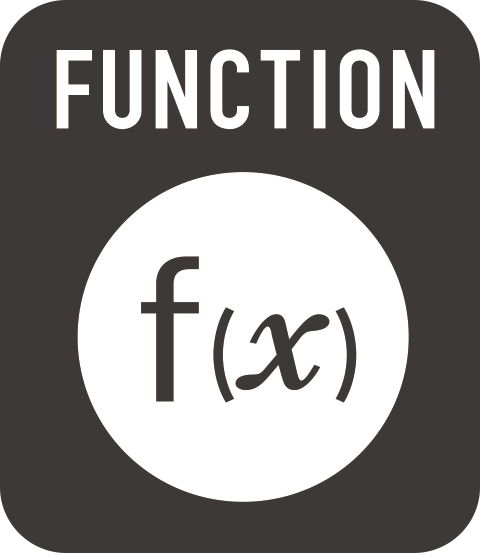#### You can store functions in both the f(x) and g(x) memory.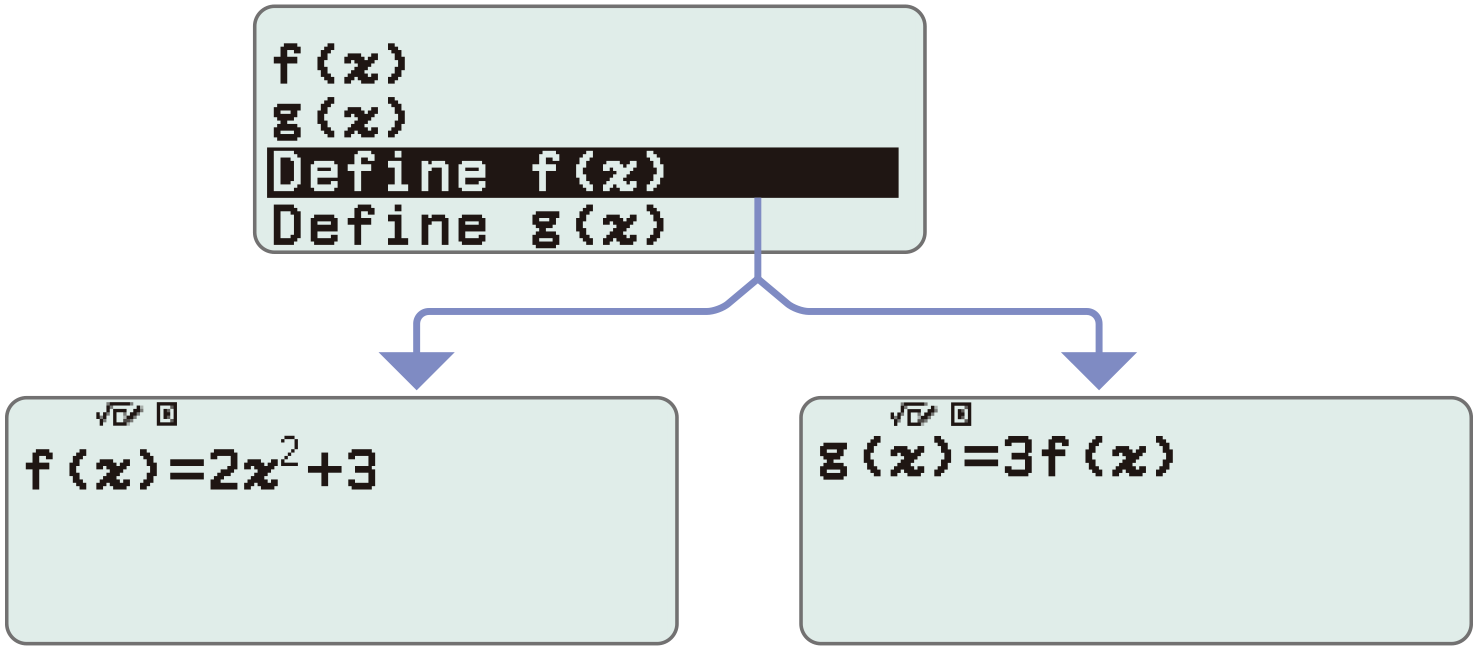#### Examples:

Create a table in the Table app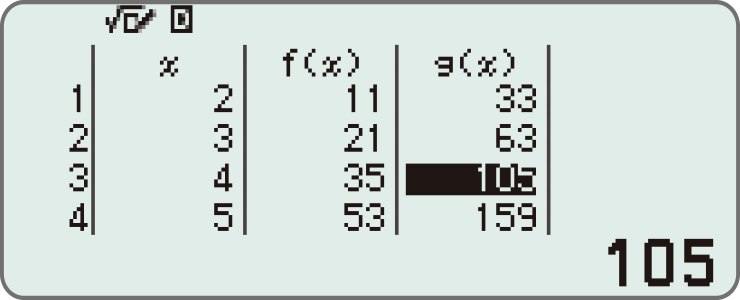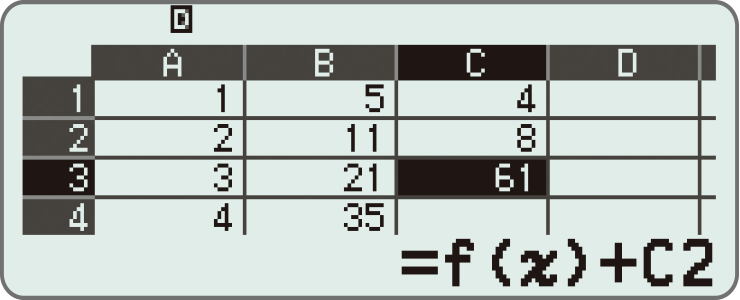#### VARIABLE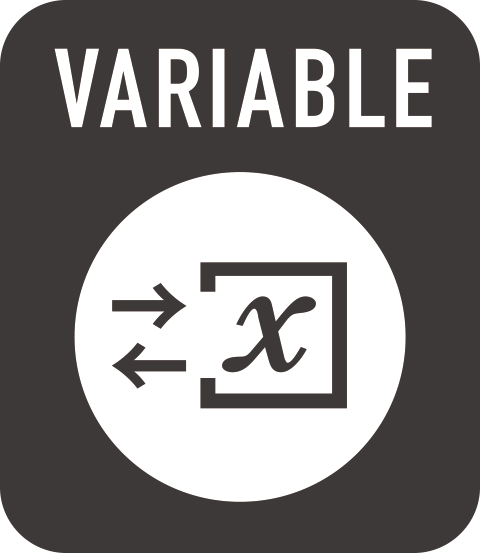#### <Things you can do with the Variable key>

Display the variable list screen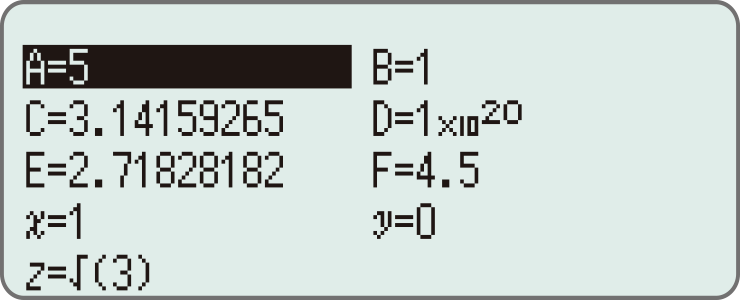Store values to variables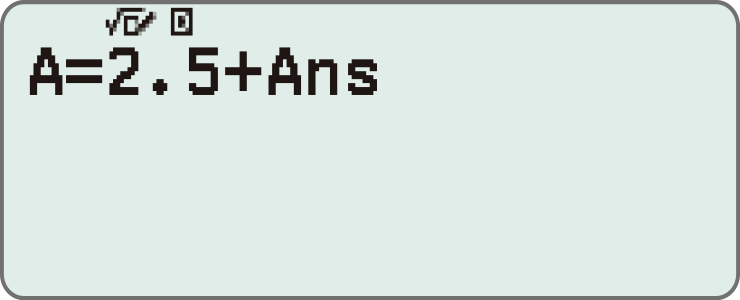Use saved values (can be called from any app)